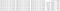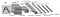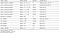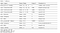# Goals

The goal of this project was to utilize deep neural networks and convolutional neural networks in order to clone driving behavior. Udacity provided a simulator application where we can steer a car around a track for data collection. The collected data is comprised of image data captured from a car’s set of cameras as well as steering angles. This is used to train a neural network and then use the model to drive the car autonomously around the track — also using the simulator.

# Repository

The final content of the project is available in my personal repository in Github.

• `drive.py`: source code that feeds the trained model to the simulator. Also used to save frames generated by simulation.
• `model.ypnb`: Jupyter notebook used for development. Contains same code as `model.py`.
• `video.py`: program that converts frames generated by `drive.py` into a MP4 video.
• `model.h5`: final trained model used to driving on Track 1
• `video_track1.mp4`: video of Track 1 simulation generated by `video.py`. Rendered from the center camera POV.
• `video_desktop.mp4` video of simulation captured by recording the computer screen.

# Collecting training data

The dataset specified in the assignment is composed by:

• the steering angle in which the car is pointing –`left > 0` and `right < 0`;
• the speed `throttle` in which the car moves.Sample excerpt from driving.log

# Model architecture

## Keras

The implementation of the convolutional neural network model for this project use the Keras which is a high-level API, that runs on top of TensorFlow.

## LeNet

LeNet is already known to us as the convolutional neural network architecture used in the German Traffic Sign Classifier project. It seemed like a good starting point as we were already familiar with it. Instead of 43 classes (or 10 from the MNIST examples) we had to adapt it to input the camera images and output the steering value.Classic LeNet Architecture

## NVIDIA

The third model to be tried was the one based on a paper published by the research team at NVIDIA. This is the network as described by the team.NVIDIA network architecture as implemented
`def model_NVIDIA():    model = Sequential()    model.add(Lambda(lambda x: x/127.5 - 1., input_shape=(64, 64, 3)))    model.add(Convolution2D(24, 5, 5, subsample=(2,2), activation='relu'))    model.add(Convolution2D(36, 5,5, subsample=(2,2), activation='relu'))    model.add(Convolution2D(48, 5, 5, subsample=(2,2), activation='relu'))    model.add(Convolution2D(64, 3, 3, activation='relu'))    model.add(Convolution2D(64, 3, 3, activation='relu'))    model.add(Flatten())    model.add(Dense(1164, activation='relu', name='FC1'))    model.add(Dense(100, activation='relu', name='FC2'))    model.add(Dense(50, activation='relu', name='FC3'))    model.add(Dense(10, activation='relu', name='FC4'))    model.add(Dense(1))    return model`

## comma.ai

Another well-known model is the one proposed by the comma.ai team. It has an architecture similar to the NVIDIA network. I built the model and tried a few runs with different parameters. However after a few iterations in which the car had trouble completing a full lap on the track I decided to abandon it since the NVIDIA model was working OK and for the sake of delivering the project under the deadline. It may require a few iterations to adjust data and the parameters to make it work.comma.ai network architecture

## Model parameter tuning

The final model used the Adam optimizer so there was no need to tune the learning rate.

# Dataset Augmentation

## Normalization

I scaled the pixel values of the data to be zero-centered, i.e. with a scale `[-1, 1]`. That is known to help neural networks to learn better and faster. As noted in the code excerpt of the NVIDIA network I chose to apply the normalization in the lambda layer of the model.

## Downsampling the set

Since the car rides forward most of the time, the dataset is heavily skewed with steering angles very close to zero. For that reason I downsampled the dataset to ignore every sample where the steering angle was lower than `0.05` in either direction (left and right).

## Side cameras

As suggested by the instructors I included the images provided from the left and right cameras in the training data. This was done at data load time by adding (or subtracting) an adjustment factor of `0.2` to the steering angle value capture with the centered camera.

`angle_center = float(sample)angle_left = angle_center + correctionangle_right = angle_center - correction`

## Image flip

Another strategy for augmenting the data is to add a mirrored version of a training camera image. This is useful because the training data is captured with a car moving in a counterclockwise direction, therefore most turns are left-bound. To provide the network with a more balanced number of left and right turns steering I included a flipped version of the image for every 2 out 3 samples. This also required the inversion of the steering angle.

`if random() > 0.666:    img = cv2.flip(img, 1)    angle = angle * -1.0`

## Processing images

Different conditions of light in the simulator can affect the trained model. Sometimes a shaded area in the track shows to be tricky, and mislead the model to think the dark area is the edge of the track. In order to minimize this I augmented the dataset by randomly changing the picture brightness. This is done by converting the image to HSV color space and then applying a random multiplier to the V channel of the image.

`def modify_brightness(img):    img = cv2.cvtColor(img,cv2.COLOR_BGR2HSV)    img = np.array(img, dtype = np.float64)    brightness_multiplier = 0.2    random_bright = brightness_multiplier + np.random.uniform()    img[:,:,2] = img[:,:,2] * random_bright    img[:,:,2][img[:,:,2]>255] = 255    img = np.array(img, dtype = np.uint8)    img = cv2.cvtColor(img, cv2.COLOR_HSV2BGR)    return img`
`img = cv2.cvtColor(img,cv2.COLOR_BGR2YUV)`

# Implementation

## Generator

My implementation uses a Python `generator` in order to generate data for training. This makes the program less likely to suffer memory issues during training as the data is never fully loaded at once in the computer memory.

## Training and Validation data

I used a ratio of 80:20 to split the data between training and validation sets.

`train_samples, validation_samples = train_test_split(samples, test_size=0.2)train_generator = generator(train_samples, batch_size)validation_generator = generator(validation_samples, batch_size)`

## Driving with the model

In order to test the trained model I used the code of `drive.py` provided by Udacity. In order to make it work I had to modify the code to include the same image augmentation methods that were used in training (i.e. color channel conversion, cropping and resizing.)

# Autonomous navigation

This is the video of a successful ride around track 1

Multi time founder, CTO and product executive. Founder @ridehugobus, EIR: @human_ventures & @techstars. VC: @collabfund

## More from Alberto Escarlate

Multi time founder, CTO and product executive. Founder @ridehugobus, EIR: @human_ventures & @techstars. VC: @collabfund